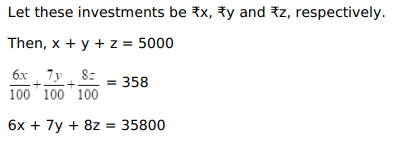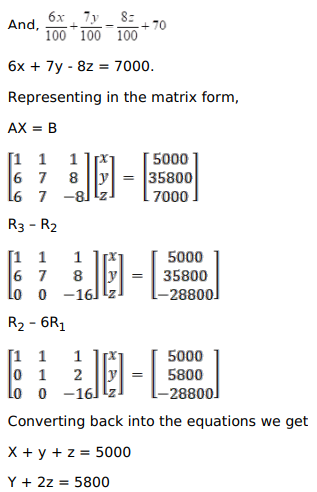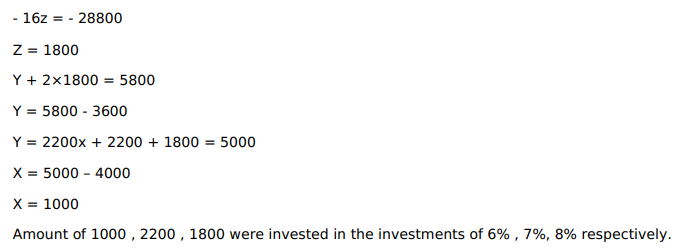# An amount of ₹ 5000 is put into three investments at

Question:

An amount of ₹ 5000 is put into three investments at $6 \%, 7 \%$ and $8 \%$ per annum respectively. The total annual income from these investments is $₹ 358$. If the total annual income from first two investments is ₹ 70 more

than the income from the third, find the amount of each investment by the matrix method.

HINT: Let these investments be ₹ $x$, ₹ $y$ and ₹ $z$, respectively.

Then, $x+y+z=5000, \ldots$ (i)

$\frac{6 x}{100}+\frac{7 y}{100}+\frac{8 z}{100}=358 \Rightarrow$

$6 x+7 y+8 z=35800$ ......(ii)

And, $\frac{6 x}{100}+\frac{7 y}{100}=\frac{8 z}{100}+70$

$\Rightarrow 6 x+7 y-8 z=7000 \ldots$ (iii)

Solution: